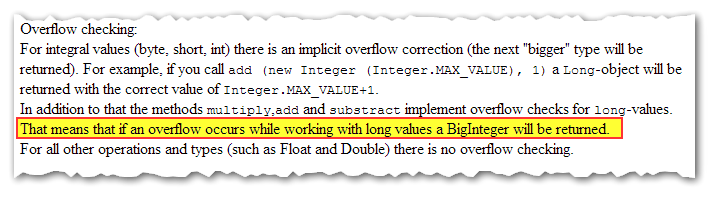For arithmetic in Velocity, the only safe approach is the so-called “tools-ish” one: use MathTool methods like $math.add, $math.sub, and $math.mul. ### The rule Aside from the one exception later in this post, you should not use the Java-like math operators (+ and -). The core reason is the plus sign + and minus sign - have different syntax rules in Velocity! You can easily create fatal errors – or worse, lines that are silently ignored – by forgetting that - must be surrounded by spaces. That’s right, only one of these is correct Velocity syntax for subtraction: #set($a = 99 )
#set( $b =$a - 1 ) ## correct
#set( $b =$a -1 )  ## fatal ParserException!
#set( $b =$a- 1 )  ## fatal ParserException!
#set( $b =$a-1 )   ## non-fatal, but doesn’t subtract!!!


So I always teach people to use #set( $b =$math.sub($a,1) ). It’s longer, but you’ll never break your code by switching sub and add. ### The exception Despite the above, in the course of developing my Base64 encoding in Velocity code, I discovered a unique requirement that could only be met by purposely using the + operator instead of $math.add.

This is the only exception I’ve ever found, and it doesn’t override my recommendation above. But it is – to me and I hope to the couple of people like me out there – fascinating.

So.

In Base64 encoding, one of the steps requires Integers to be converted to binary Strings, 42 → “00101010”. (If this is complete gibberish to you, you can reread this post when you have a couple more years of development under your belt!)

To get those Integers, you need to convert signed Bytes (-128 through 127) to their equivalent all-positive Integers (0 through 255).

To convert signed Bytes, you need to use a bitwise AND. But the traditional AND operator, the & symbol in Java and most other languages, isn’t available in Velocity.

Since you can’t use notation like #set( $c =$a & $b ) in Velocity (that’s a fatal error) you need to find a method-based way – a “tools-ish” way, somewhat ironically – to do a bitwise AND: something like #set($c = $a.and($b) ).

#### MathUtils vs. MathTools

Hidden in the doc for Velocity’s MathUtils (part of Velocity’s internal API, not for public consumption) I found this gem:So now we know Velocity can do – in fact is explicitly documented to do – exactly what we need. If we add 1 to the max value of a Long, the result will be a BigInteger (and will also have the correct value, obviously).

Except this only applies to the literal + operator. MathTools’ $math.add also does addition, but doesn’t implement the “overflow correction” logic. Let’s compare. Using the + operator: #set($Integer = 0 )
Integer $Integer.class.getName() =$Integer
#set( $Long =$field.in($Integer).MAX_VALUE + 1 ) Long$Long.class.getName() = $Long #set($BigInteger = $field.in($Long).MAX_VALUE + 1 )
BigInteger $BigInteger.class.getName() =$BigInteger


The output will be:

Integer java.lang.Integer = 0
Long java.lang.Long = 2147483648
BigInteger java.math.BigInteger = 9223372036854775808


Exactly what we need. $BigInteger is a BigInteger we can use as a BigInteger factory ($BigInteger.valueOf) and then do fancy stuff like .and() and .or() and .xor() which would otherwise be impossible in Velocity.

Let’s try using $math.add: #set($Integer = 0 )
Integer $Integer.class.getName() =$Integer
#set( $Long =$math.add($field.in($Integer).MAX_VALUE,1) )
Long $Long.class.getName() =$Long
#set( $BigInteger =$math.add($field.in($Long).MAX_VALUE,1) )
BigInteger $BigInteger.class.getName() =$BigInteger


The output will be:

Integer java.lang.Integer = 0
Long java.lang.Long = 2147483648
BigInteger java.lang.Long = 9223372036854775807


Whoa. We attempted to add 1 to the largest Long, but the attempt silently failed. $BigInteger remains a Long, and it never gets incremented: it’s still Long.MAX_VALUE or 9,223,372,036,854,775,807. ### It’s notable, but doesn’t change the rule The likelihood that you’d otherwise need to perform BigInteger-range arithmetic within Marketo is basically zero. I mean, it would have to be something like… you’re using a webhook to take the squares of lead scores, and you have a score in the millions and must raise it to the power of 2 in a Velocity token. Let’s face it, relative to the chances you will at some point forget the spaces around -, that’s just not gonna happen. The risk/reward overwhelmingly favors $math.add and \$math.sub.

In this case we needed a BigInteger not because of its infinite range of values, but because we need to get at the cool methods it has – which Integers and Longs do not. (The underlying value is tiny, between -128 and 127 then converted to between 0 and 255.) So for this particular purpose, deliberately using + makes sense.